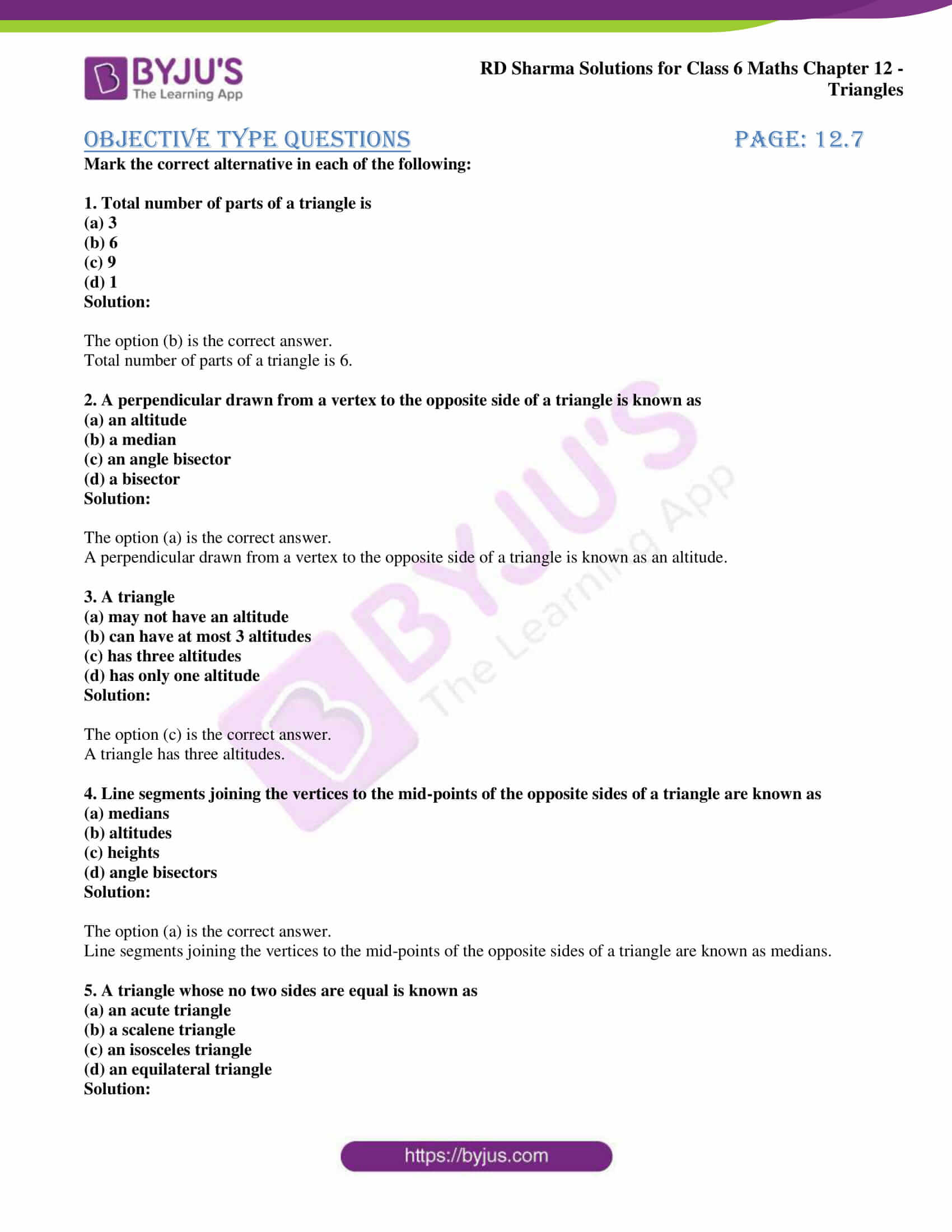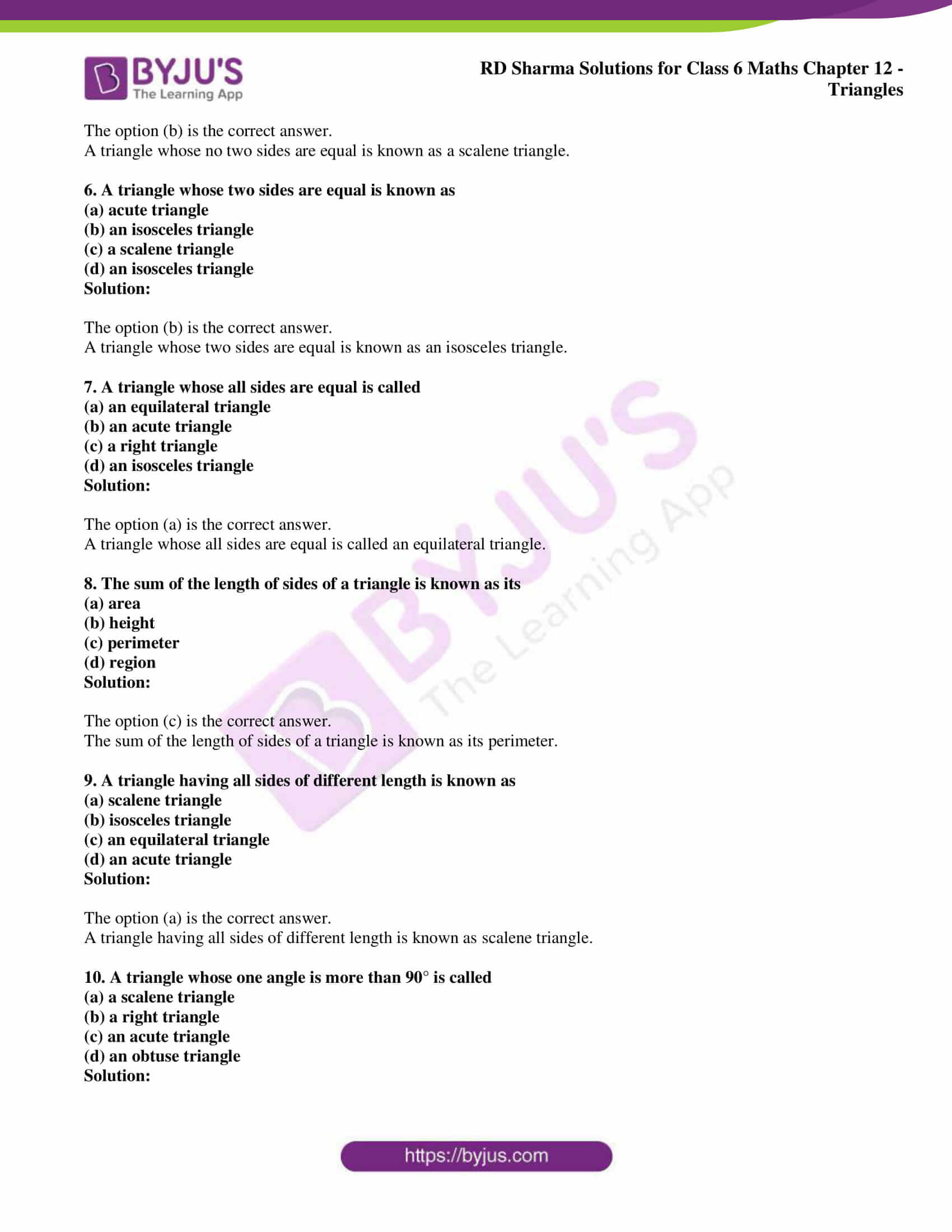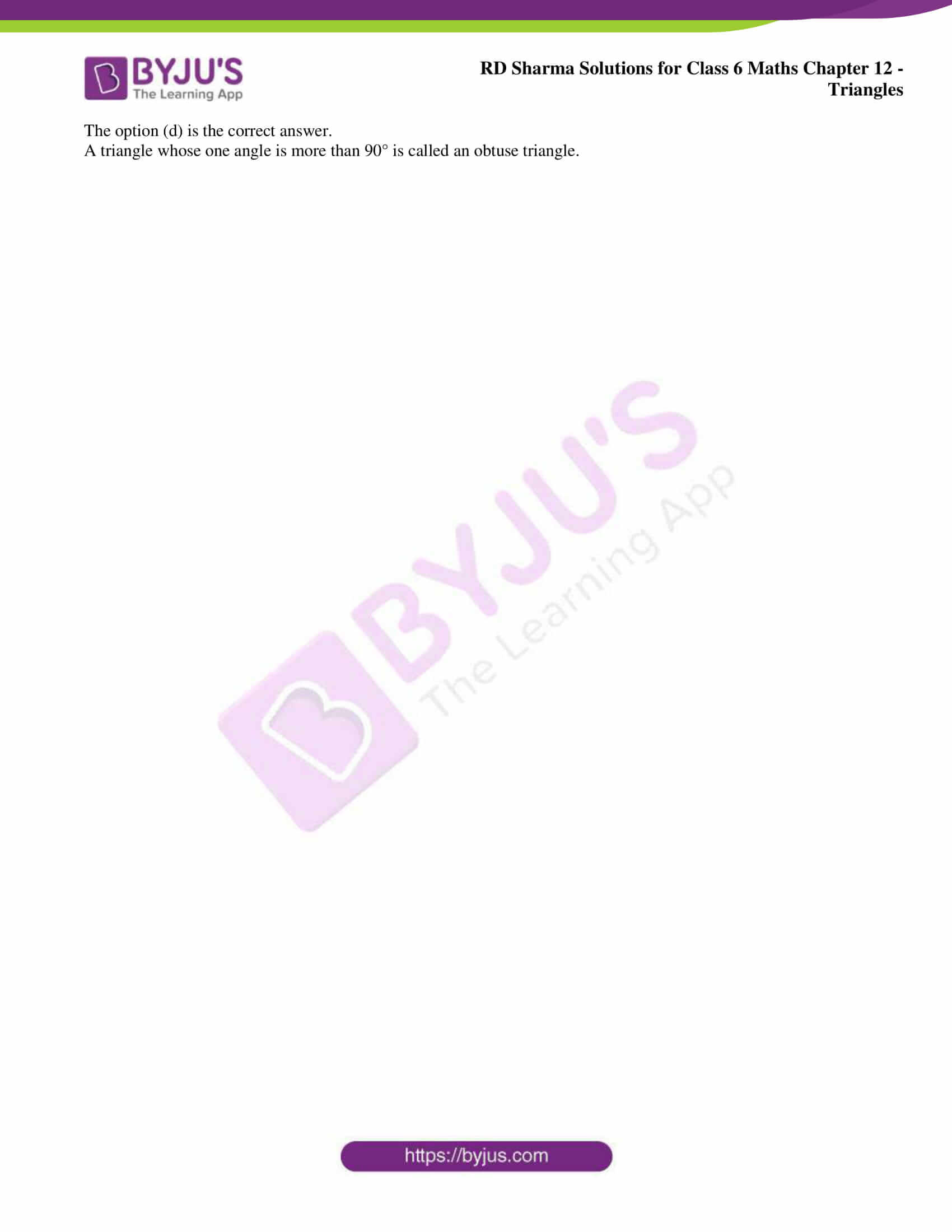# RD Sharma Solutions for Class 6 Maths Chapter 12: Triangles Objective Type Questions

The students can self analyse their areas of weaknesses by practising problems using solutions prepared by experts. The objective type questions help students glance the entire chapter at one stretch and to get a hold on various concepts which are covered here. The regular practice of problems using the solutions improves problem-solving abilities which are important from the exam point of view. The students can use RD Sharma Solutions Class 6 Maths Chapter 12 Triangles Objective Type Questions PDF which are provided here to solve exercise wise problems.

## RD Sharma Solutions for Class 6 Maths Chapter 12: Triangles Objective Type Questions Download PDF### Access answers to Maths RD Sharma Solutions for Class 6 Chapter 12: Triangles Objective Type Questions

Mark the correct alternative in each of the following:

1. Total number of parts of a triangle is
(a) 3
(b) 6
(c) 9
(d) 1

Solution:

The option (b) is the correct answer.

Total number of parts of a triangle is 6.

2. A perpendicular drawn from a vertex to the opposite side of a triangle is known as
(a) an altitude
(b) a median
(c) an angle bisector
(d) a bisector

Solution:

The option (a) is the correct answer.

A perpendicular drawn from a vertex to the opposite side of a triangle is known as an altitude.

3. A triangle
(a) may not have an altitude
(b) can have at most 3 altitudes
(c) has three altitudes
(d) has only one altitude

Solution:

The option (c) is the correct answer.

A triangle has three altitudes.

4. Line segments joining the vertices to the mid-points of the opposite sides of a triangle are known as
(a) medians
(b) altitudes
(c) heights
(d) angle bisectors

Solution:

The option (a) is the correct answer.

Line segments joining the vertices to the mid-points of the opposite sides of a triangle are known as medians.

5. A triangle whose no two sides are equal is known as
(a) an acute triangle
(b) a scalene triangle
(c) an isosceles triangle
(d) an equilateral triangle

Solution:

The option (b) is the correct answer.

A triangle whose no two sides are equal is known as a scalene triangle.

6. A triangle whose two sides are equal is known as
(a) acute triangle
(b) an isosceles triangle
(c) a scalene triangle
(d) an isosceles triangle

Solution:

The option (b) is the correct answer.

A triangle whose two sides are equal is known as an isosceles triangle.

7. A triangle whose all sides are equal is called
(a) an equilateral triangle
(b) an acute triangle
(c) a right triangle
(d) an isosceles triangle

Solution:

The option (a) is the correct answer.

A triangle whose all sides are equal is called an equilateral triangle.

8. The sum of the length of sides of a triangle is known as its
(a) area
(b) height
(c) perimeter
(d) region

Solution:

The option (c) is the correct answer.

The sum of the length of sides of a triangle is known as its perimeter.

9. A triangle having all sides of different length is known as
(a) scalene triangle
(b) isosceles triangle
(c) an equilateral triangle
(d) an acute triangle

Solution:

The option (a) is the correct answer.

A triangle having all sides of different length is known as scalene triangle.

10. A triangle whose one angle is more than 90° is called
(a) a scalene triangle
(b) a right triangle
(c) an acute triangle
(d) an obtuse triangle

Solution:

The option (d) is the correct answer.

A triangle whose one angle is more than 90° is called an obtuse triangle.